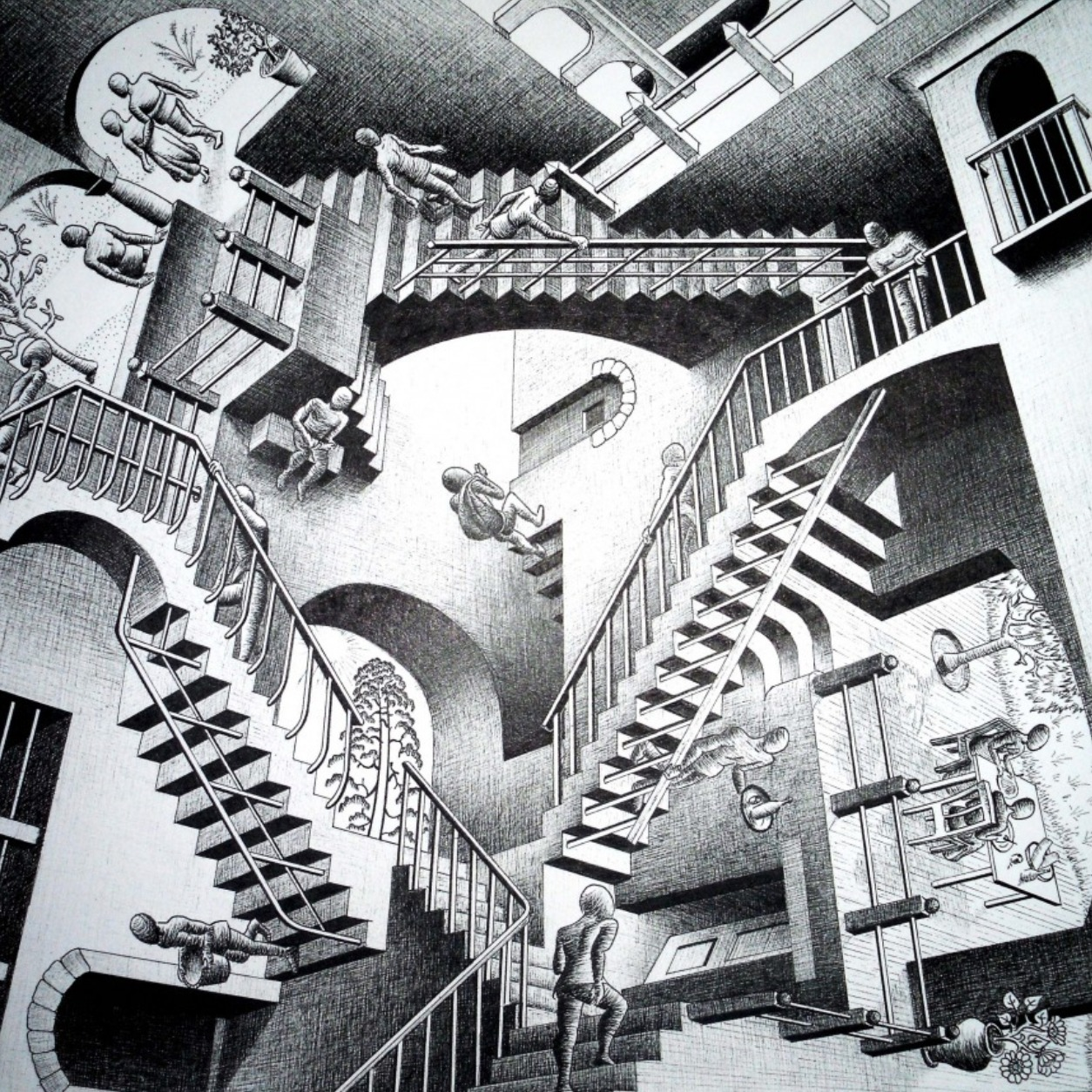# Multiplicativityof sets

Number theory

Expanding the known multiplicative properties of large difference sets yields a new, quantitative proof on the structure of product sets.

## On some multiplicative properties of large difference sets

In press Canadian Journal of Mathematics (2023)

I. Shkredov

In our paper we study multiplicative properties of difference sets $A−A$ for large sets $A \subseteq \mathbb{Z}/q\mathbb{Z}$ in the case of composite $q$. We obtain a quantitative version of a result of A. Fish about the structure of the product sets $(A−A)(A−A)$. Also, we show that the multiplicative covering number of any difference set is always small.

In press Canadian Journal of Mathematics (2023)

I. Shkredov